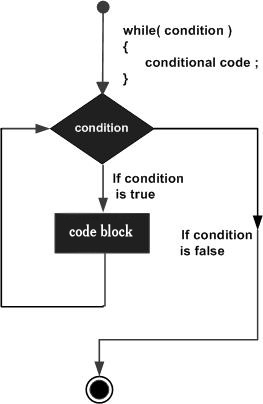while循环在D编程语言循环语句多次，只要给定的条件为真时，可执行一个目标语句多次。

## 语法

`while(condition) { statement(s); }`

## 流程图：## 例如：

```import std.stdio;

int main ()
{
/* local variable definition */
int a = 10;

/* while loop execution */
while( a < 20 )
{
writefln("value of a: %d", a);
a++;
}

return 0;
}```

```value of a: 10
value of a: 11
value of a: 12
value of a: 13
value of a: 14
value of a: 15
value of a: 16
value of a: 17
value of a: 18
value of a: 19```